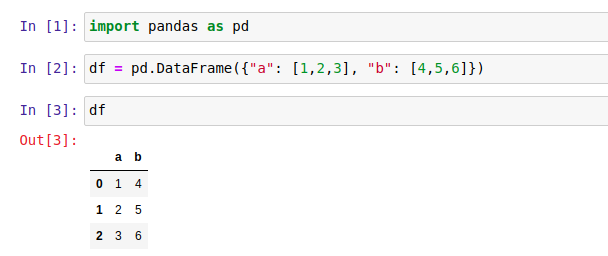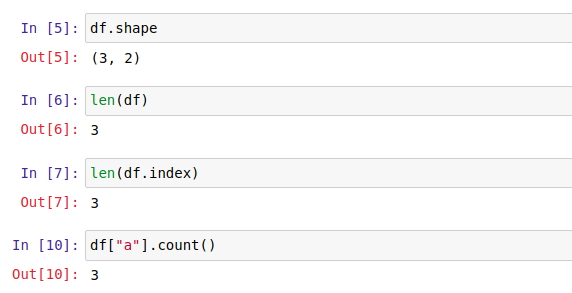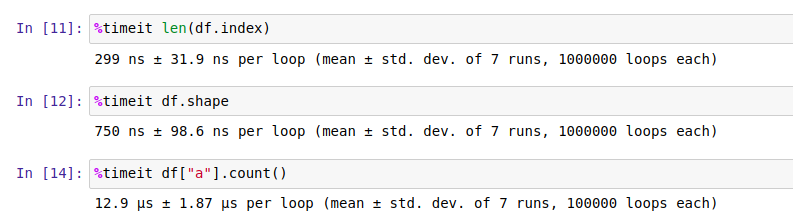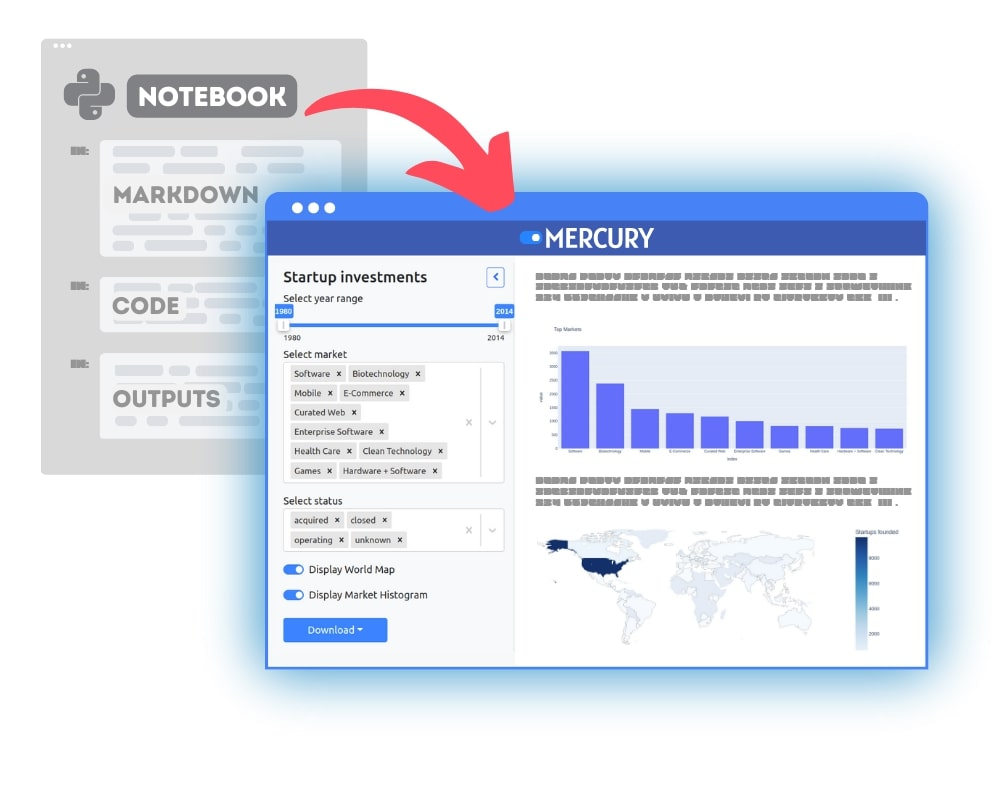The Pandas it’s a popular data manipulation library. The Pandas has over 15k stars on Github. It’s an open-source project that allows, among others: automatic and explicit data alignment, easy handling of missing data, Intelligent label-based slicing, indexing, and subsetting of large data sets, merging data sets, or flexible reshaping and pivoting of data sets There are 3 ways to get the row count from Pandas DataFrame. I will describe them all in this article. My preferred way is to use `df.shape` to get number of rows and columns. This method is fast and simple.

## 1. `df.shape`

Let’s create a simple DataFrame:

``````import pandas as pd

df = pd.DataFrame({"a": [1,2,3], "b": [4,5,6]})
``````

The notebook view:The simplest approach to get row count is to use `df.shape`. It returns the touple with a number of rows and columns:

``````nrows, ncols = df.shape
``````

If you would like to get only the number of rows, you can try the following:

``````nrows, _ = df.shape

# or

nrows = df.shape
``````

## 2. `len(df)`

The fastest approach (slightly faster than `df.shape`) is just to call `len(df)` or `len(df.index)`. Both approaches return the DataFrame row count, the same as the `index` length.

``````nrows = len(df)

# or

nrows = len(df.index)
``````

## 3. `df[df.columns].count()`

We can use `count()` function to count a number of not null values. We can select the column by name or using `df.columns` list:

``````nrows = df["a"].count()

# or

nrows = df[df.columns].count()
``````

It is the slowest method because it counts non-null values.

Below is the image with the code for all three methods:## Performance

I’ve compared the performance of methods using `timeit` magic command in Jupyter Notebook.

The fastest approach is to use `len(df.index)`. The slowest approach is to count non-null values with `count()`.## Summary

Padas DataFrame is a great way to manipulate data (small or large). My preferred way is to use `df.shape`. The method is speedy and additionally provides information about a number of columns.### Convert Python Notebooks to Web Apps

We are working on open-source framework Mercury for converting Jupyter Notebooks to interactive Web Applications.### Articles you might find interesing